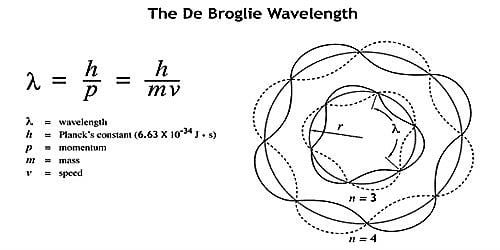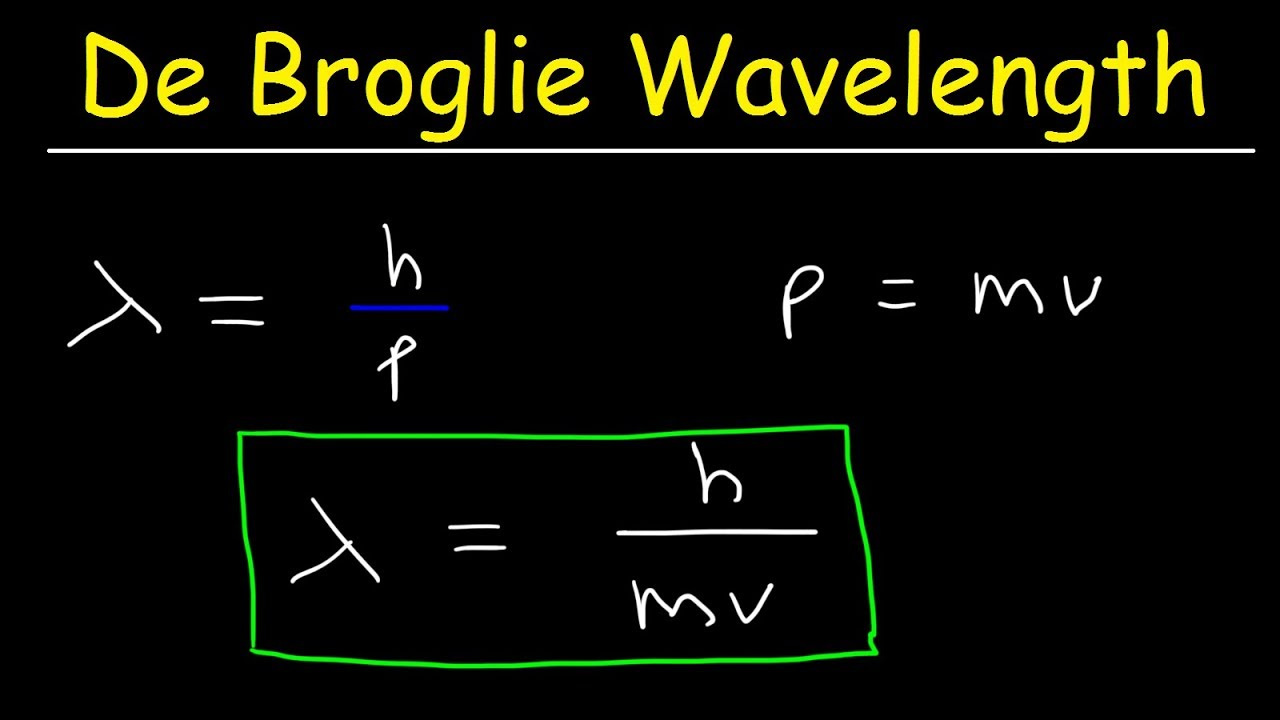# De Broglie theory (Duality)

The photoelectric effect showed that light consists of streams of particles called photons. This leads to the conclusion that like matter, light radiation can sometimes behave as particles. The phenomenon of interference, diffraction and polarization of the light indicate that light is wave. Light therefore, exhibits dual nature. It behaves like particle as well as wave. De Broglie thought nature loves symmetry and argued if light can behave as waves in some situation and as particles in other situation, then why cannot particles behave like waves. Electrons and other materials particles can therefore show wave properties.

Statement:

The wave properties is associated with each particle and wavelength associated with the particle is given by

λ = h/mv ……………………….. (i)

Where ‘h’ is planck’s constant, m is the mass of particles and v is the velocity of the particles.

Equation (i) is known as De Broglie wave equation

Since Planck’s constant is very small, the De Broglie wave is negligible to microscopic level where mass of the particle is very large. Therefore, it is most common in the microscopic level or atomic level.Image source: QSStudy

Derivation of De Broglie wavelength

According to planck the energy of wave of frequency ν is given by,

E = hν ……………………………… (ii)

Let a particle of mass has the same energy as a photon (i.e., E = h ν)

According to Einstein’s equation for a particle

E = mc2 ………………………………  (iii)

From the equation (ii) and equation (iii) we get

hv = mc2

or,  hc/λ   = mc2    ( since, c = νλ)

or,  λ = h/mc

For the particle moving with velocity v,

λ = h/mv

This is De Broglie wave equationApplication of De Broglie wave theory

Some of the applications of this theory are:

A) Root of atomic structure

The De -Broglie wave theory was the root of atomic structure. This theory gave the concept that the electron in an orbit remains at standing wave. This theory supports the Bohr’s Postulate.

Let ‘λ’ be the wavelength associated with an electron moving in an orbit of radius r around the nucleus.

In a stable orbit, the circumference of the orbit must be equal to an integral multiole of wavelength, so

2πr λ

2 π r = nλ

2 π r = nh/mv (∵λ = h/mv)

Or, mvr = nh/2π

B) Electron microscope

• The De –Broglie wave theory has led to the development of an electron microscope.
• This microscope, due to its larger magnification is highly suitable for the study of atomic structure.
• Two years after the announcement of the “De –Broglie wave theory Davison and Germer (in 1927) demonstrated the wave associated with the electrons.

References: